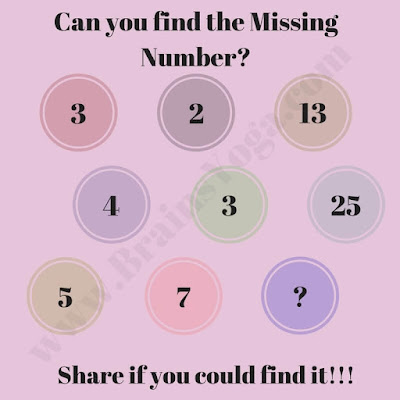This is very interesting missing number picture puzzle in which some group of numbers are given in Circles. These numbers are related to each other with some Mathematical series or pattern. Can you find this series or pattern and then calculate the value of missing number which will replace the question mark?Can you find the missing number?